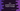# C++ program to print Floyd's triangle using for and while loop# Introduction :

In this C++ tutorial, we will learn how to print Floyd’s triangle programmatically. Floyd triangle is named after Robert Floyd. It is a right angled triangle created by using natural numbers. It looks like as below :

``````1
2	3
4	5	6
7	8	9	10
11	12	13	14	15
``````

The first row has 1 number, the second row has 2, the third row has 3 etc. The numbers are starting from 1 and increase by 1 on each step if we iterate the triangle one by one row and from left to right.

Our program will take the height of the triangle as an input from the user. With this tutorial, you will learn how to use loops, how to print values, how to read values in C++.

## C++ program using for loop :

``````#include <iostream>
using namespace std;
int main()
{
//1
int height;
int value = 1;
//2
cout << "Enter the height of the triangle : " << endl; cin >> height;
cout << "\n"
<< "Floyd's triangle of height " << height << " :"
<< "\n"
<< endl;
//3
for (int i = 0; i < height; i++)
{
//4
for (int j = 0; j <= i; j++)
{
cout << value << " ";
value++;
}
cout << endl;
}
return 0;
}``````

## Explanation :

The commented numbers in the above program denote the step numbers below :

1. Create one integer variable height to store the height of the triangle and value to indicate the current value to print.

2. Ask the user to enter the height of the triangle. We are using cout to print a message to the user. Read that value and store it in the variable height. cin is used for reading a value.

3. Now, run two for loops. The outer one will run from i = 0 to i = height - 1. e.g if the value of height is 4, it will run from i = 0 to i = 3 i.e. 4 times. The inner loop will run from j = 0 to j = i. e.g. if the value of i is 2, it will run for 2 times.

The outer loop is used to indicate each row of the triangle and the inner loop is used to indicate each column of a row in the triangle.

1. Inside both loops, print the value of the variable value. Also, increment it by 1. This variable is initialized as 1. So it will print the numbers serially on each step like 1, 2, 3, 4, 5….

### Sample Output :

``````Enter the height of the triangle :
5

Floyd's triangle of height 5 :

1
2 3
4 5 6
7 8 9 10
11 12 13 14 15

Enter the height of the triangle :
8

Floyd's triangle of height 8 :

1
2 3
4 5 6
7 8 9 10
11 12 13 14 15
16 17 18 19 20 21
22 23 24 25 26 27 28
29 30 31 32 33 34 35 36
``````

## Floyd’s triangle using a while loop in C++ :

We can also use one while loop to print the same Floyd’s triangle :

``````#include <iostream>
using namespace std;
int main()
{
int height;
int value = 1;
cout << "Enter the height of the triangle : " << endl; cin >> height;
cout << "\n"
<< "Floyd's triangle of height " << height << " :"
<< "\n"
<< endl;
int i = 0, j;
while (i < height)
{
j = 0;
while (j <= i)
{
cout << value << " ";
value++;
j++;
}
cout << endl;
i++;
}
return 0;
}``````

The output is the same as like above.

## Conclusion :

In this tutorial, you have learned how to print Floyd’s triangle or a number right angled triangle in C++ using for loop and while loop. Try to go through the program and drop one comment below if you have any questions.